# Summing Positive and Negative Numbers Separately

In this article, we are going to learn how to calculate the sum of positive and negative numbers separately on the basis of criteria. We will use SUMIF function to sum up the positive and negative numbers.

Let’s take an example and understand:-

We have an agency’s car sales data. In data we have sales details along with discount detail. Now, we want to calculate the sum for every manufacturer sales amount and discount amount. Also, in data, discount amount is in negative numbers so we want to return the total sum of negative number as well.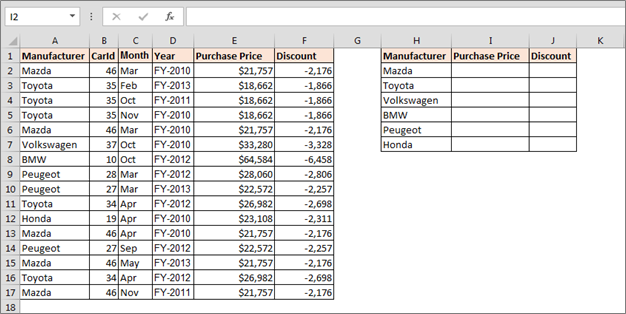How to calculate the sum of positive numbers?

To calculate the positive numbers, we will use SUMIF function, follow below given steps:-

• Enter the formula in cell I2
• =SUMIF(\$A\$2:\$F\$17,H2,\$E\$2:\$E\$17)
• Press Enter and copy the same formula in range.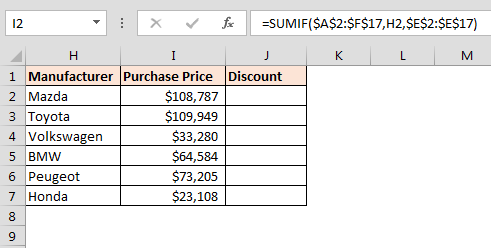To calculate the sum of negative numbers, we will use SUMIF function. We need to follow below given steps:-

• Enter the formula in cell J2
• =SUMIF(\$A\$2:\$F\$17,H2,\$F\$2:\$F\$17)
• Press Enter and copy the same formula in range.
• When we use the formula (-) sign will be deleted.
• Press Ctrl+1 format cells, and a dialog box will appear.
• Format Cells > Numbers > Custom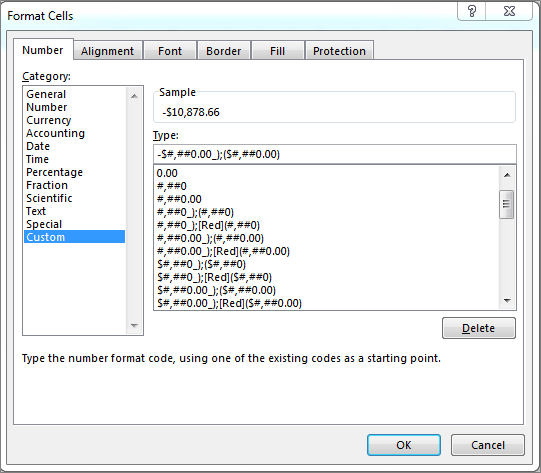• Click on OK.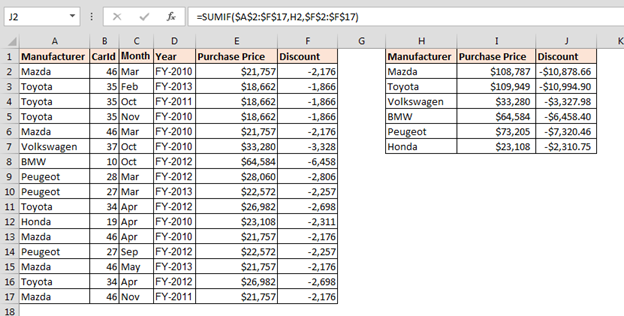This is the way we can use SUMIF to calculate the negative and positive number in the data of Microsoft Excel.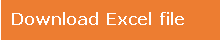1.Hermann Kreimer

I understand the way you sum the positive and negative numbers if they are in two separate columns.
But what if all numbers are in the same column?

•Hi Harmann,

Let's say you have those numbers in range A2:A10.
To sum only positive numbers from range A2:A10 the formula is =SUMIF(A2:A10,">=0")
To sum only negative numbers from range A2:A10 the formula is =SUMIF(A2:A10,"<0")

Terms and Conditions of use

The applications/code on this site are distributed as is and without warranties or liability. In no event shall the owner of the copyrights, or the authors of the applications/code be liable for any loss of profit, any problems or any damage resulting from the use or evaluation of the applications/code.